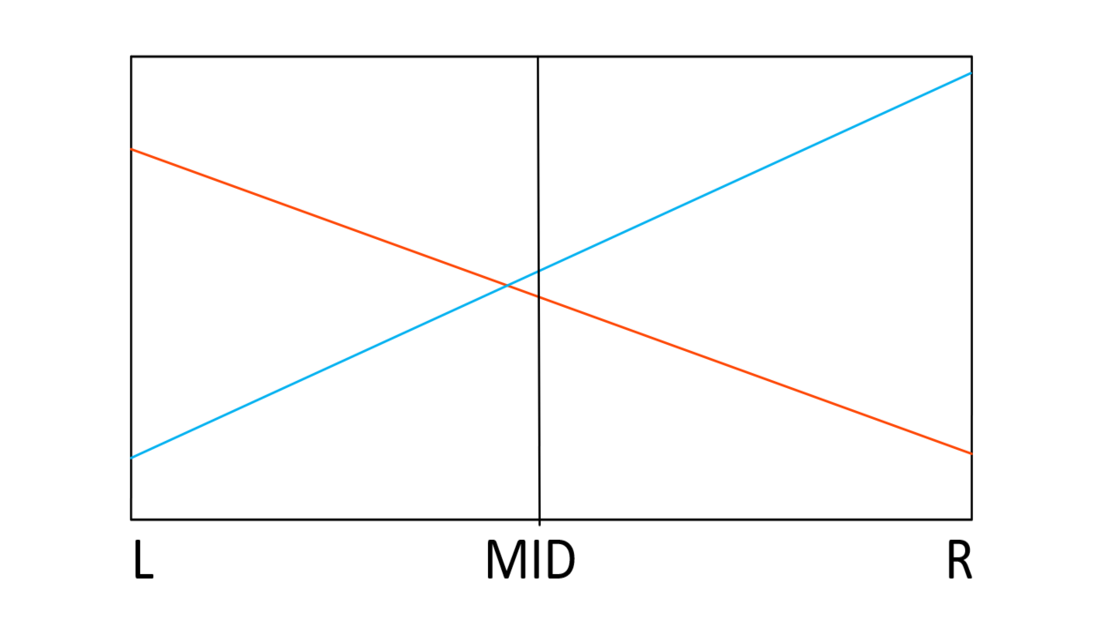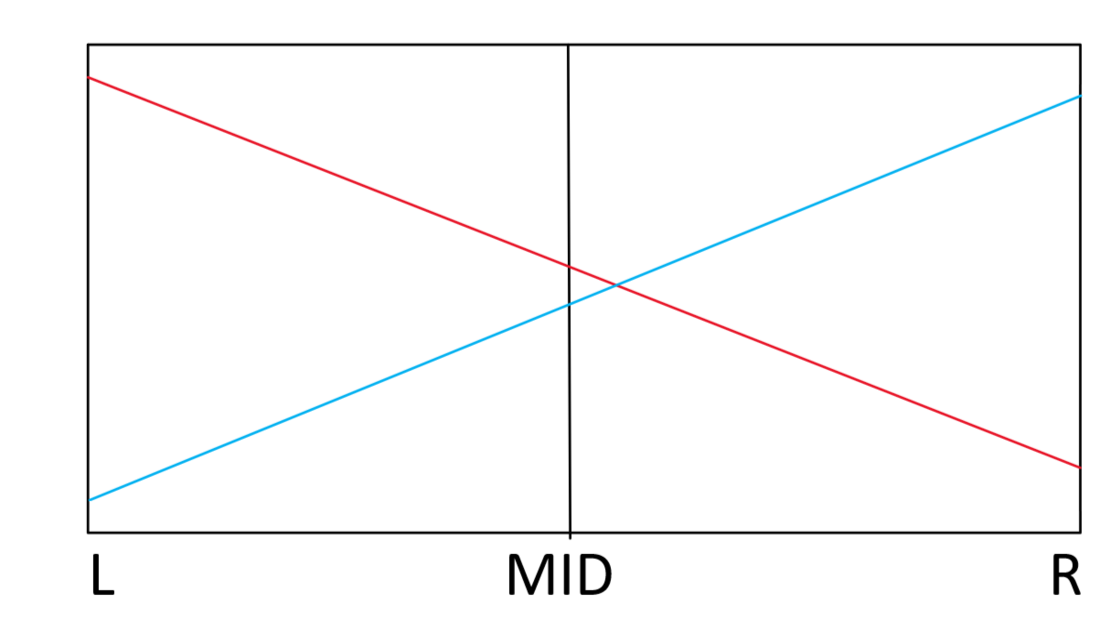Open in App
Not now

# Introduction to Li Chao Tree

• Difficulty Level : Hard
• Last Updated : 14 Mar, 2023

A Li Chao tree (also known as a Dynamic Convex Hull or Segment Tree with lazy propagations) is a data structure that allows for efficient dynamic maintenance of the convex hull of a set of points in a 2D plane. The Li Chao tree allows for dynamic insertion, deletion, and query operations on the set of points, and can be used in a variety of geometric problems such as line segment intersection, visibility graphs, and polygon triangulation.

• The structure of a Li Chao tree is a segment tree, a balanced binary tree data structure where each leaf node represents a single point, and each internal node represents a convex hull of the points represented by its child nodes.
• The tree is balanced to ensure that each leaf node is at roughly the same depth, which allows for logarithmic time complexity for operations such as insertion and deletion.

To go further one should begin with some geometric utility functions as given below:

## C++

 struct line {     long double m, b;       // This overloads the () operator,     // allowing the struct to be used as a     // function that takes in a value x and     // returns the result of m * x + b.     long double operator()(long double x)     {           return m * x + b;     } };   vector a(N * 4);

## Java

 class Line {     double m, b;           public Line(double m, double b) {         this.m = m;         this.b = b;     }           public double evaluate(double x) {         return m * x + b;     } }   List a = new ArrayList<>(N * 4);

## Python3

 class Line:     def __init__(self, m, b):         self.m = m         self.b = b     def __call__(self, x):         return self.m * x + self.b   a = [Line(0,0) for i in range(N * 4)]

## C#

 public class Line {     private double m;     private double b;     public Line(double m, double b)     {         this.m = m;         this.b = b;     }       public double Evaluate(double x) {            return m * x + b;      } }   List a = new List(N * 4);

On every node of the segment tree, we are storing the line that maximizes (or minimizes) the value of the mid. If the interval of the node is [L, R), then the line stored in it will maximize(or minimize).Insert: Let’s say we are inserting a  new line to the node which is corresponding to Interval [L, R).To make it easy let’s assume that the line on the node has a smaller slope. So, mid =\ frac{L + R}{2}.  Now we have two cases to think about.

Note: Red is the original line in the node

Case 1: red(mid) < blue(mid)

In this Case, We have to replace red line with blue.Now, should we remove red?  The answer is No, because there is need of red in segment [L, mid). Because of this reason we should pass red to the node with interval [L, mid), which is its left son.representation of case 1

Case 2: red(mid) > blue(mid)

In similar way, we should pass blue to its right son and keep red in this node, whose interval is [mid, R).representation of case 2

Below is the code for the above approach:

Code block

## C++

 void insert(int l, int r, line segment, int idx = 0) {     // if the range represented by the current node is just     // one element     if (l + 1 == r) {         // if the segmentment to be inserted has a greater         // slope than the one in the current node, replace         // it         if (segment(l) & gt; a[idx](l))             a[idx] = segment;         return;     }     // find the middle of the range     int mid = (l + r) / 2;     // calculate the indices of the left and right child     // nodes     int leftson = idx * 2 + 1, rightson = idx * 2 + 2;     // if the segmentment to be inserted has a smaller slope     // than the one in the current node, swap them     if (a[idx].m > segment.m)         swap(a[idx], segment);     // if the segmentment in the current node has a smaller     // value at the middle point than the segmentment to be     // inserted     if (a[idx](mid) < segment(mid)) {         // swap the segmentments in the current node and the         // left child node         swap(a[idx], segment);         // insert the segmentment into the left child node         insert(l, mid, segment, leftson);     }     // otherwise, insert the segmentment into the right     // child node     else         insert(mid, r, segment, rightson); }

Code for queries, as we know we only need to consider the intervals that contain the point we need to ask from the way we inserted lines.

## C++

 long double query(int l, int r, int x, int idx = 0) {     // If the range of the query is only     // one element, return the result of     // the function a at index o applied to x     if (l + 1 == r)         return a[idx](x);       // Find the middle of the range     int mid = (l + r) / 2;     // Find the index of the left and right     // children of the current node     int leftson = idx * 2 + 1;     int rightson = idx * 2 + 2;       // If the value being queried is less     // than the middle of the range,     // recursively call query on     // the left child     if (x < mid)         return max(a[idx](x), query(l, mid, x, leftson));       // Otherwise, recursively call query     // on the right child     else         return max(a[idx](x), query(mid, r, x, rightson)); }

## Python3

 def query(l, r, x, idx = 0):     # If the range of the query is only     # one element, return the result of     # the function a at index o applied to x     if l + 1 == r:         return a[idx](x)       # Find the middle of the range     mid = (l + r) // 2           # Find the index of the left and right     # children of the current node     leftson = idx * 2 + 1     rightson = idx * 2 + 2       # If the value being queried is less     # than the middle of the range,     # recursively call query on     # the left child     if x < mid:         return max(a[idx](x), query(l, mid, x, leftson))       # Otherwise, recursively call query     # on the right child     else:         return max(a[idx](x), query(mid, r, x, rightson))         # This code is contributed by ik_9

Time Complexity: O(NLogN), for the construction of the tree and O(LogN) for each Query
Auxiliary Space: O(N)

### Advantages of Li Chao tree:

•   Dynamic Convex Hull: The Li Chao tree is a dynamic data structure that can handle line segments and can be used to maintain the convex hull of a set of lines in real-time.
•   Fast Queries: Li Chao tree has a logarithmic time complexity for querying the minimum value on a given line segment, making it efficient for large datasets.
•   Space-efficient: Li Chao tree uses a balanced binary tree structure, which is space-efficient.

### Disadvantages of the Li Chao tree:

•   Complexity: The construction of the Li Chao tree takes O(n log n) time and O(n) space, which can be computationally expensive for large datasets.
•   Limited to lines: Li Chao tree can only handle line segments and not other types of geometric shapes.
•   Limited to 2D: Li Chao tree is limited to 2-dimensional space and cannot be used for 3D or higher-dimensional problems.
•   Limited to linear functions: Li Chao tree is limited to linear functions and cannot be used for non-linear functions.

My Personal Notes arrow_drop_up
Related Articles